# Loops in Java (for, while, do-while)

Challenge Inside! : Find out where you stand! Try quiz, solve problems & win rewards!
Video Tutorial## Overview

Looping is a feature that facilitates the execution of a set of instructions repeatedly until a certain condition holds false. Java provides three types of loops namely the for loop, the while loop, and the do-while loop. Loops are also known as Iterating statements or Looping constructs in Java.

## Introduction to Loops in Java

Imagine you are asked to print the value of $2^1$, $2^2$, $2^3$, $2^4$ …….$2^{10}$. One way could be taking a variable $x=2$ and printing and multiplying it with 2 each time.

Output:

Another way is using loops. Using Looping statements the above code of 10 lines can be reduced to 3 lines.

Output:

Explanation:

Looping Constructs in Java are statements that allow a set of instructions to be performed repeatedly as long as a specified condition remains true.

Java has three types of loops i.e. the for loop, the while loop, and the do-while loop. for and while loops are entry-controlled loops whereas do-while loop is an exit-controlled loop.

A very obvious example of loops can be the daily routine of programmers i.e. Eat -> Sleep -> Code -> Repeat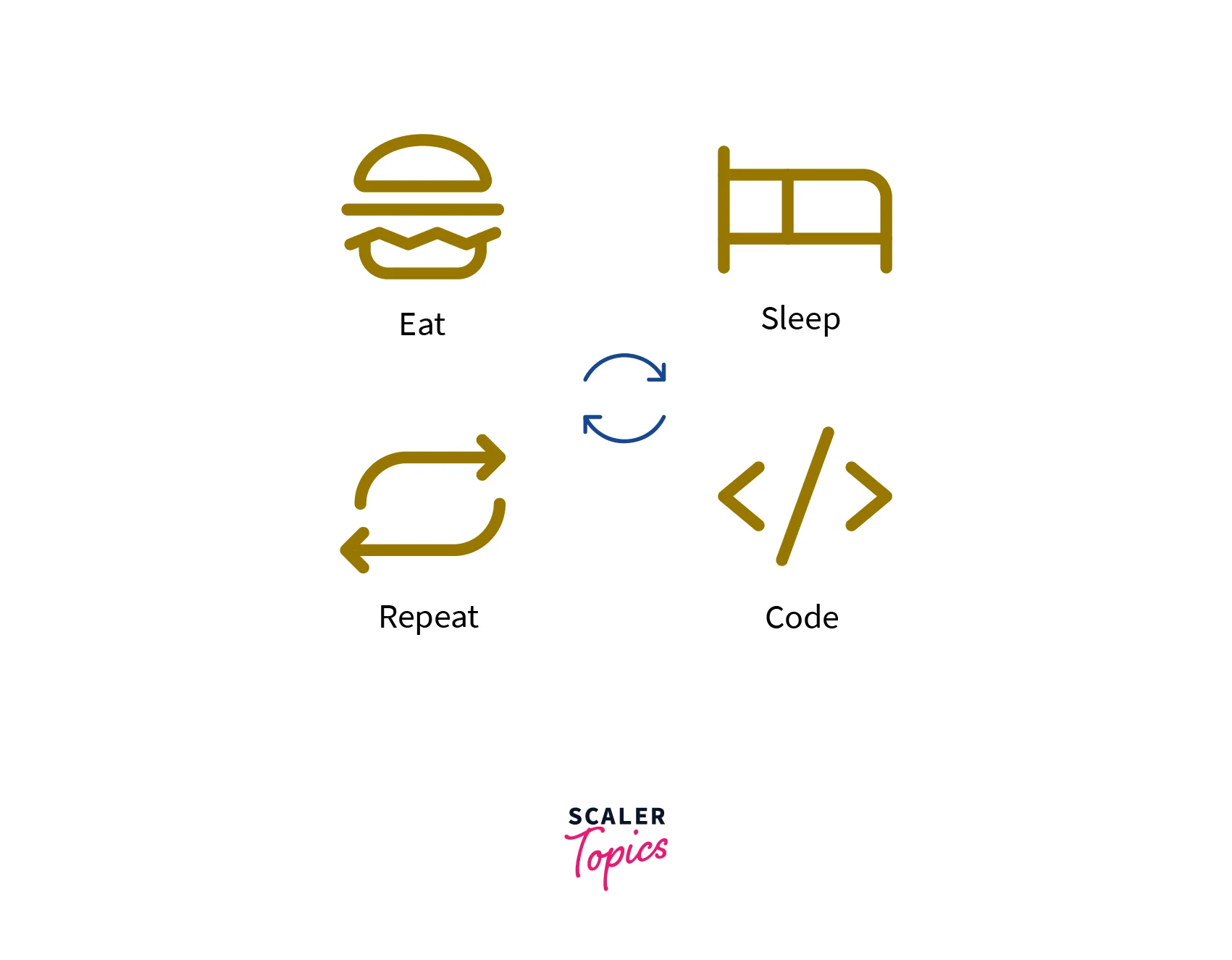## Need for Looping Constructs in Java

A computer is most suitable for performing repetitive tasks and can tirelessly do tasks tens of thousands of times. We need Loops because

• Loops facilitates 'Write less, Do more' - We don't have to write the same code again and again.
• They reduce the size of the Code.
• Loops make an easy flow of the control.
• They also reduce the Time Complexity and Space Complexity - Loops are faster and more feasible as compared to Recursion.

## Elements of the Loops in Java

1. Initialization Expression(s): Initialization is carried out only once before entering the loop. Here, we either declare and initialize a control variable(s) or only initialize the variable(s) we are going to use in looping. We can initialize multiple variables as well.
2. Test Expression (Condition): It is a boolean expression. Its value decides whether the loop will be executed or terminated. If the condition is satisfied, the control goes inside the loop, otherwise, it is terminated. In an exit-controlled loop, the test expression is evaluated before exiting from the loop whereas in an entry-controlled loop the test expression is evaluated before entering the loop.
3. Body of the Loop: The statements that are to be executed repeatedly are written in the body of the Loop. They are executed until the condition of the loop is true.
4. Update Expression(s): Here, we update the value of the loop variable(s). It is used so that after some point of time the loop terminates. It is executed at the end of the loop when the loop-body has been executed and the next iteration is to start. It is also called Increment/Decrement expression since in most of the cases value of loop variables is either incremented or decremented.

To understand all these steps let us relate this to our day-to-day lives. We wake up every morning and think of the tasks that we are going to accomplish in a day. This is just like initializing.

We test several possibilities, like availability of resources, pre-planned events, etc., to check whether we can complete the tasks. For example, to attend online classes we check for a good internet connection. This can be related to test expressions.

Then we complete the tasks, (eat-sleep-code) as in the body of the loop. And the next day we repeat the same process.

As Sunday arrives we take a break from the routine, just like that when certain test condition fails, we come out of the loop.

Note

Update expression doesn’t need to be increment or decrement only. It can be any update to the variable x. For example, i+=2, i*=3, or i/=5 etc.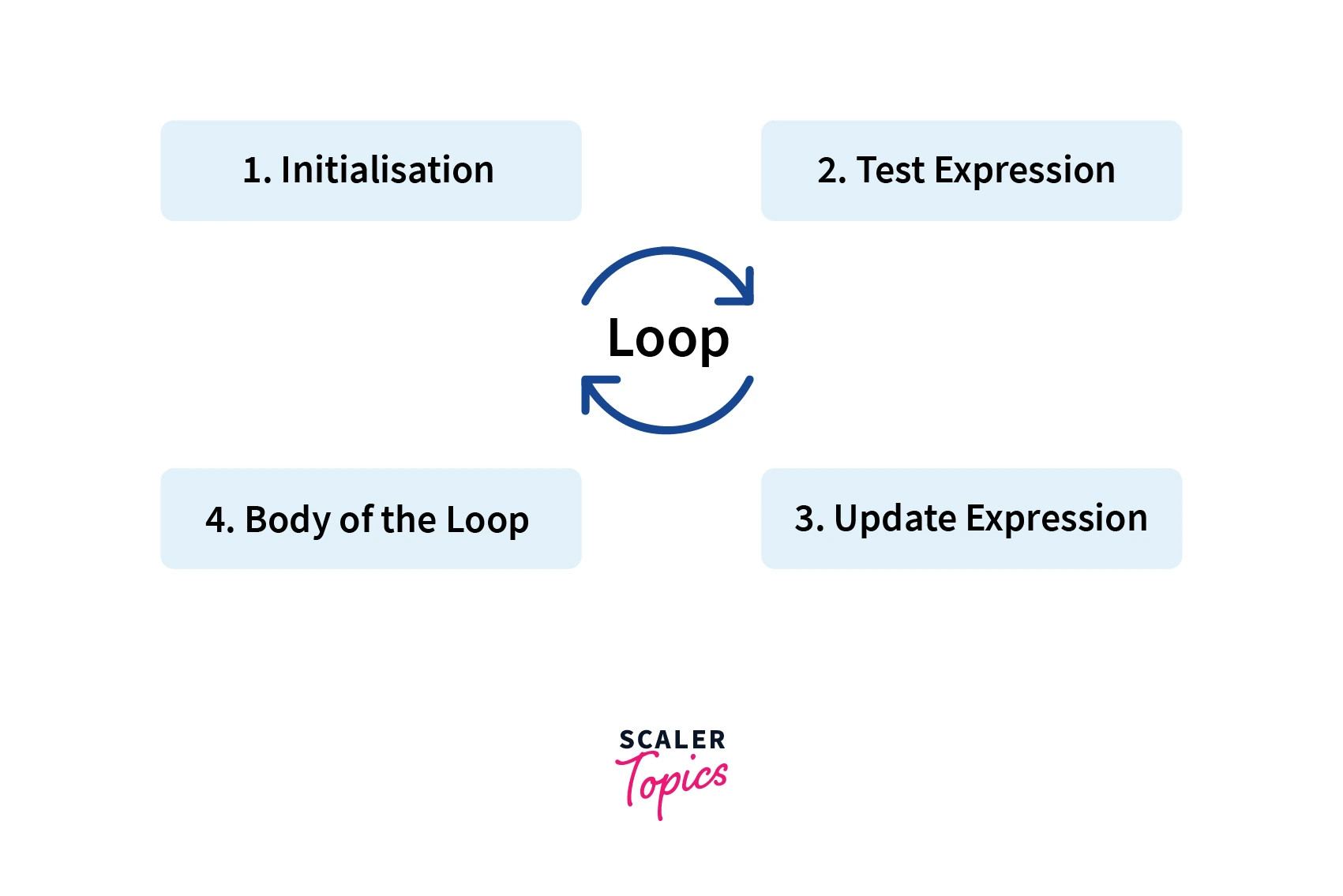## Types of Loops in Java

There are three types of Loops. They are:

1. for Loop
2. while Loop
3. do-while Loop

## for Loop

When we know the exact number of times the loop is going to run, we use for loop. It provides a concise way of writing initialization, test condition, and increment/decrement statements in one line. Thus, it is easy to debug and also has no risk of forgetting any part of the loop, since the condition is checked before.

For loop is an entry-controlled loop as we check the condition first and then evaluate the body of the loop.

Syntax:

Example

Output:

Explanation:

Here, initially i is 1 which is less than maxNum so it is printed. Then i is incremented and printed until it is greater than maxNum.

Explanation:

IterationVariableCondition i<=maxNumAction and Update Expression
1sti=1, maxNum=5truePrint 1 and Increment i
2ndi=2, maxNum=5truePrint 2 and Increment i
3rdi=3, maxNum=5truePrint 3 and Increment i
4thi=4, maxNum=5truePrint 4 and Increment i
5thi=5, maxNum=5truePrint 5 and Increment i
6thi=6, maxNum=5falseTerminate Loop

### Working of a for Loop

Firstly Initialization expression is executed. Then the test expression is evaluated. If it is true then control goes inside the body of the loop, otherwise, loop is terminated. After execution update expression is carried out. If the test expression evaluates to false the loop is terminated else the body of the loop is again executed. This process continues until the test expression becomes false. If the test expression never becomes false, it is the case of an infinite loop.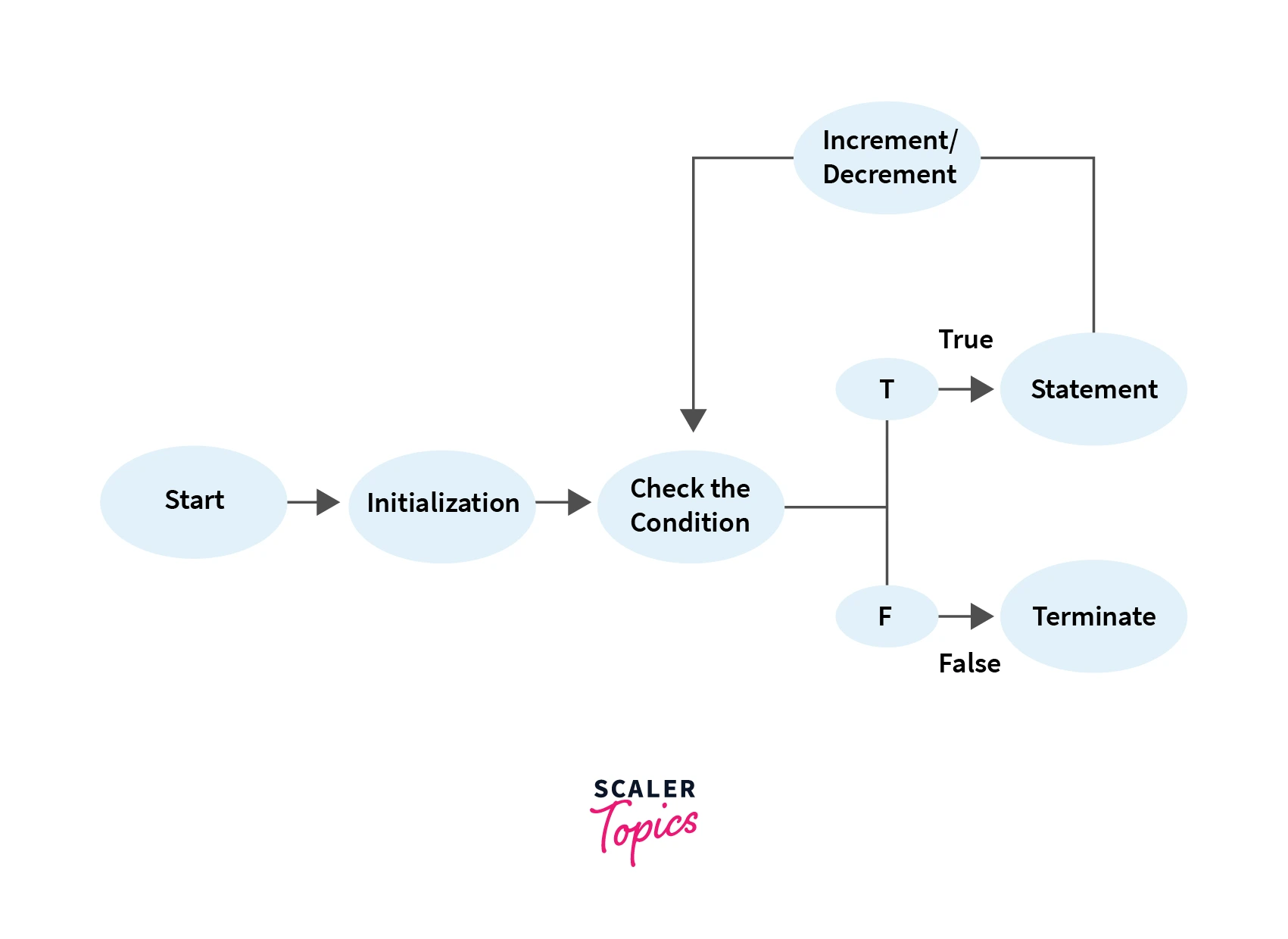### for Loop Variations

1. Multiple Initializations and Update Expressions

The for loop can have multiple initialization expressions and/or update expressions. Imagine you have a 2D matrix and you want to fill only diagonal places with 1. You need two variables i and j to represent the row index and column index in the matrix. Using these two values, a cell can be uniquely identified in the matrix. In this example, you can initialize two variables, check two conditions and update two variables in a single loop.

Note: Here, for initialization and update expressions we used commas but for conditions we used ‘&&’ (and) operator. Other Logical operators can also be used.

Output

Explanation:

In the above example, row and col are two variables that are initialized simultaneously in the initialization expression. And both of them are updated in the update expression to get the desired index for the matrix.

2. Optional Expressions

In loops, initialization expression(s), test expression(s), and update expression(s) are optional. Any or all of these are skippable.

Examples:

Let's see an example where initialization is done outside the loop, condition checking and update expression are performed inside the loop.

Output:

Explanation:

Thus, all these elements of the loop are optional. The loop-control expressions in a for loop statements are optional, but semi-colons must be written.

3. Infinite Loop

An infinite loop is a looping construct that executes the loop body infinitely until the user manually stops the execution. It is also called an endless loop or indefinite loop. It doesn't terminate at all.

Elements of the for loop are optional but while skipping them, special care must be taken. Many times due to logical mistakes we run into an infinite loop that is not desired.

For instance,

Here, i is supposed to be greater than or equal to zero and it is incremented by one each time. The condition is going to be true for all the values of i and thus the loop will run infinitely.

Another Example could be where there is no condition provided.

Infinite loops are useful in operating systems, servers, etc. They keep on executing until shut down manually.

4. Empty Loop

An empty loop is a loop that doesn’t have a body or has an empty body. It has applications too. It is used to delay execution by introducing a costly loop that does nothing.

It is different from the infinite loop as in infinite loop condition is true throughout and the loop may have a body, whereas the empty loop runs for a large but finite number of times and it doesn’t have a body.

For Example:

5. Enhanced for Loop in Java

• The Java for-each loop or enhanced for loop is introduced since J2SE 5.0. It provides an alternative approach to traverse the array or collection in Java. It is mainly used to traverse the array or collection elements.
• The advantage of the for-each loop is that it eliminates the possibility of bugs and makes the code more readable. It is known as the for-each loop because it traverses each element of the given collection one by one.
• The drawback of the enhanced for loop is that it cannot traverse the elements in reverse order. Here, we do not have the option to skip any element because it does not work on an index basis i.e. we cannot access the index of elements, the loop only returns elements.

Syntax:

Example:

Explanation:

In the given program for-each loop takes each element one by one from the nums array and adds it to the sum.

## while Loop

The while loop is used when the number of iterations is not known but the terminating condition is known. Loop is executed until the given condition evaluates to false. while loop is also an entry-controlled loop as the condition is checked before entering the loop. The test condition is checked first and then the control goes inside the loop.

Although for loop is easy to use and implement, there may be situations where the programmer is unaware of the number of iterations, it may depend on the user or the system. Thus, when the only iterating and/or terminating condition is known, a while loop is to be used.

While loop is much like repeating if statement.

Syntax:

Example Let us look at an example where we find the factorial of a number. So, until i is not equal to 0, while loop will be executed, and i will be multiplied to variable fact.

The factorial of a number n is given as $n = n*(n-1)*(n-2).....*1$. Here, until the number is not zero, the factorial variable is multiplied by the number and decremented.

Explaination

ifactConditionAction
51Truefact = 5, i = 4
45Truefact = 20, i = 3
320Truefact = 60, i = 2
260Truefact = 120, i = 1
1120Truefact = 120, i = 0
0120FalseLoop Terminated

### Working of a while Loop

Firstly, the test expression is checked. If it evaluates to true, the statement is executed and again condition is checked. If it evaluates to false the loop is terminated.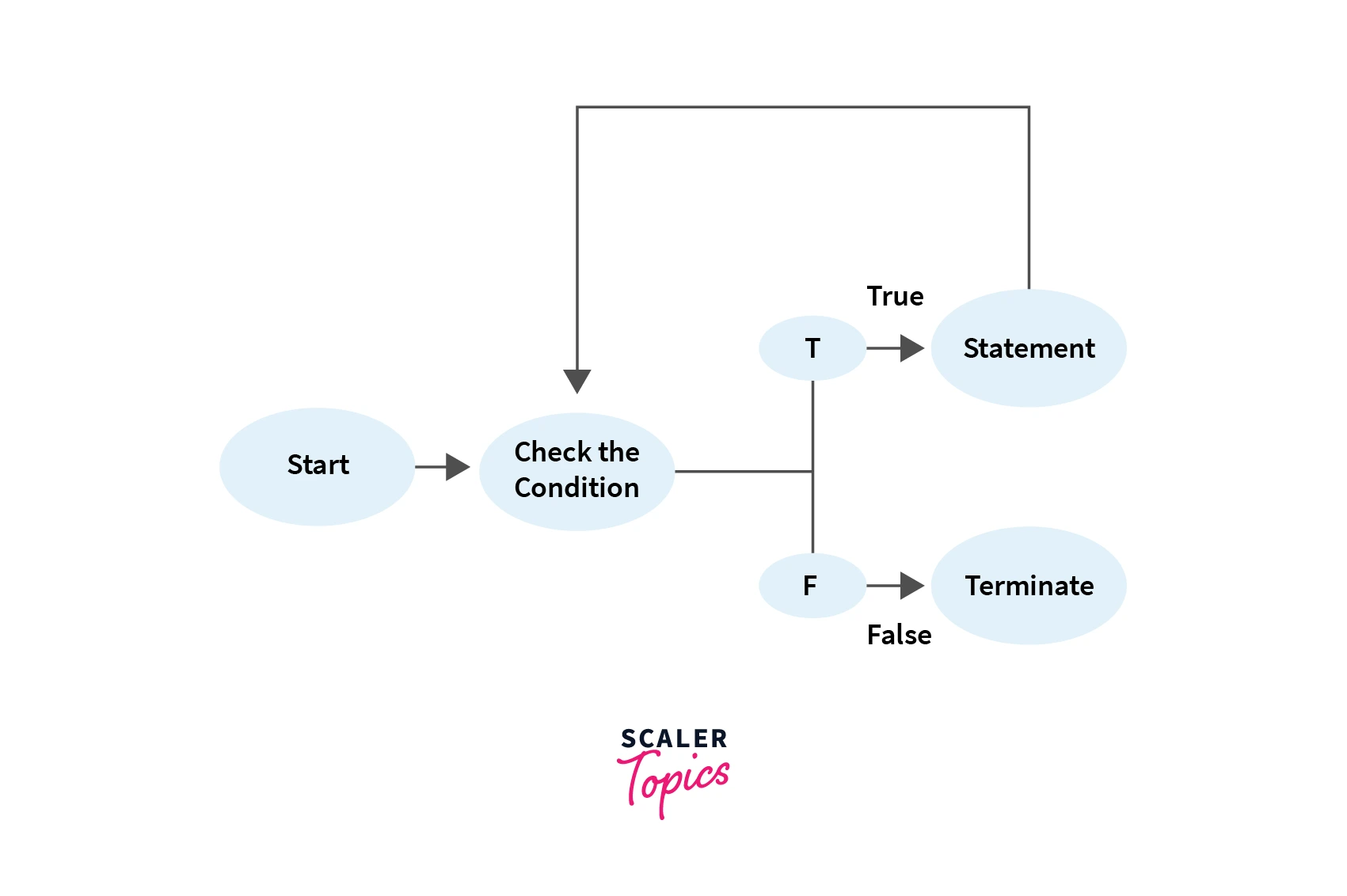### While Loop Variations

1. Empty while Loop

The empty while loop doesn't contain any statement in its body. It behaves as an infinite loop if the initial condition is satisfied.

Explanation:

The loop runs infinitely as the initial condition is satisfied and there is no update operation.

2. Infinite while Loop

An infinite while loop is a very common error made by programmers where often they forget to update statements and the loop runs infinite times.

In the above example, the value of j is not decremented and thus the loop is executed infinite times.

Also sometimes while loop is made to run infinite times as per the requirement of the program by giving the condition to be true as shown in the example.

## do-while Loop

The do-while loop is like the while loop except that the condition is checked after evaluation of the body of the loop. Thus, the do-while loop is an example of an exit-controlled loop.

The loop is executed once, and then the condition is checked for further iterations. This loop runs at least once irrespective of the test condition, and at most as many times the test condition evaluates to true. It is the only loop that has a semicolon(;).

Need for do-While Loop: The instructions inside for and while loops are not evaluated if the condition is false. In some cases, it is wanted that the loop body executes at least once irrespective of the initial state of test expression. This is where the do-while loop is used.

Syntax:

Example:

Output:

Explanation:

The above program takes two numbers from the user and performs operations on them. The program runs at least once and at max as many times, as the user wants to continue.

It first takes a number initially. It then asks for another number and operation to be performed with them. It prints the result and asks the user if they want to continue.

If they enter 1, the body of the do-while loop executes again.

### Working of a do-while Loop

The body of do-while loop is executed once no matter what the initial condition is. Then the condition is checked. If it is true then the loop is executed else it is terminated. Condition is checked at the end of the execution of the body.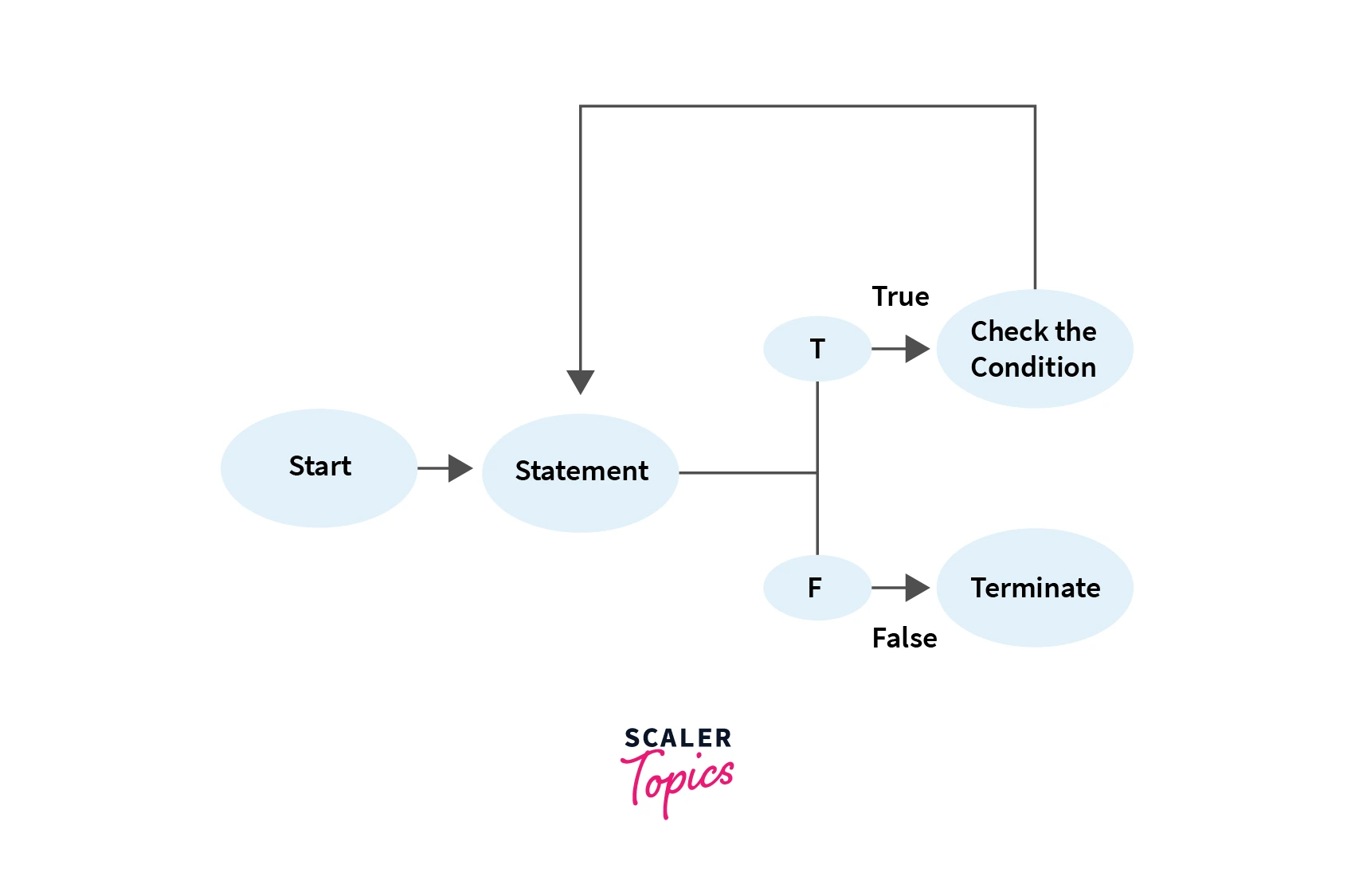## Declaration of Variables inside the Loop

The variables declared inside the loops we have discussed so far, are called local variables and their scope is only inside the loop. They cannot be accessed outside the loop. They are created and discarded each time the loop is executed.

For instance

Output:

## Nested Loops in Java

A nested loop is a loop statement inside another loop statement. In a nested loop, for each iteration of the outer loop, the entire inner loop is executed.

For instance, if the outer loop is to be run m times and the inner loop is to be run n times, the total time complexity of the nested loop is m*n i.e. for all m iterations of the outer loop, the inner loop is run n times.

Loops can be nested with the same type of loop or another type of loop there is no restriction on the order or type of loop. For example for loop can be inside a while loop and vice versa, or a do-while loop can be under another do-while loop.

Example:

One of the most common uses of nested loops are used to print multidimensional arrays, patterns, etc.

Pascals's Triangle using nested loops:

Output:

Explanation:

This function uses nested loops. The outer for loop keeps the record of rows. Two inner loops are responsible for printing spaces and the value of ^n^Cr of the rows and the number.

The first for loop prints space from 0 to totalRows - rows. And the second for loop prints the value of ^n^Cr of the current row and current number. The number is incremented each time the inner loop iterates but the value of rows remains the same. rows changes when both the loop iterates and terminates. Now the rows is incremented and again both the inner loops are again executed.

## Comparison of Loops in Java

• Though looping constructs in Java are very flexible and can be used in almost all situations yet there are some situations where one loop fits better than the other.
• The for loop is appropriate when you know in advance how many times the loop will be executed, i.e., the upper limit on the number of iterations is already known.
• The other two loops while and do-while loops are more suitable in situations when the user is only aware of the terminating condition but the number of times the loop is going to run is not known.
• The while and for loops should be preferred when you may not want to execute the loop body even once, in case the test condition is false, and the do-while loop should be preferred when you're sure you want to execute the loop-body at least once even if the test condition fails.
• These are not strict rules. The choice of a loop should depend upon the point that the loop should make our program the easiest to follow and clearest to understand.
• For and while loops are entry-controlled loops and do-while is an exit-controlled loop.
• Syntax:
forwhiledo-while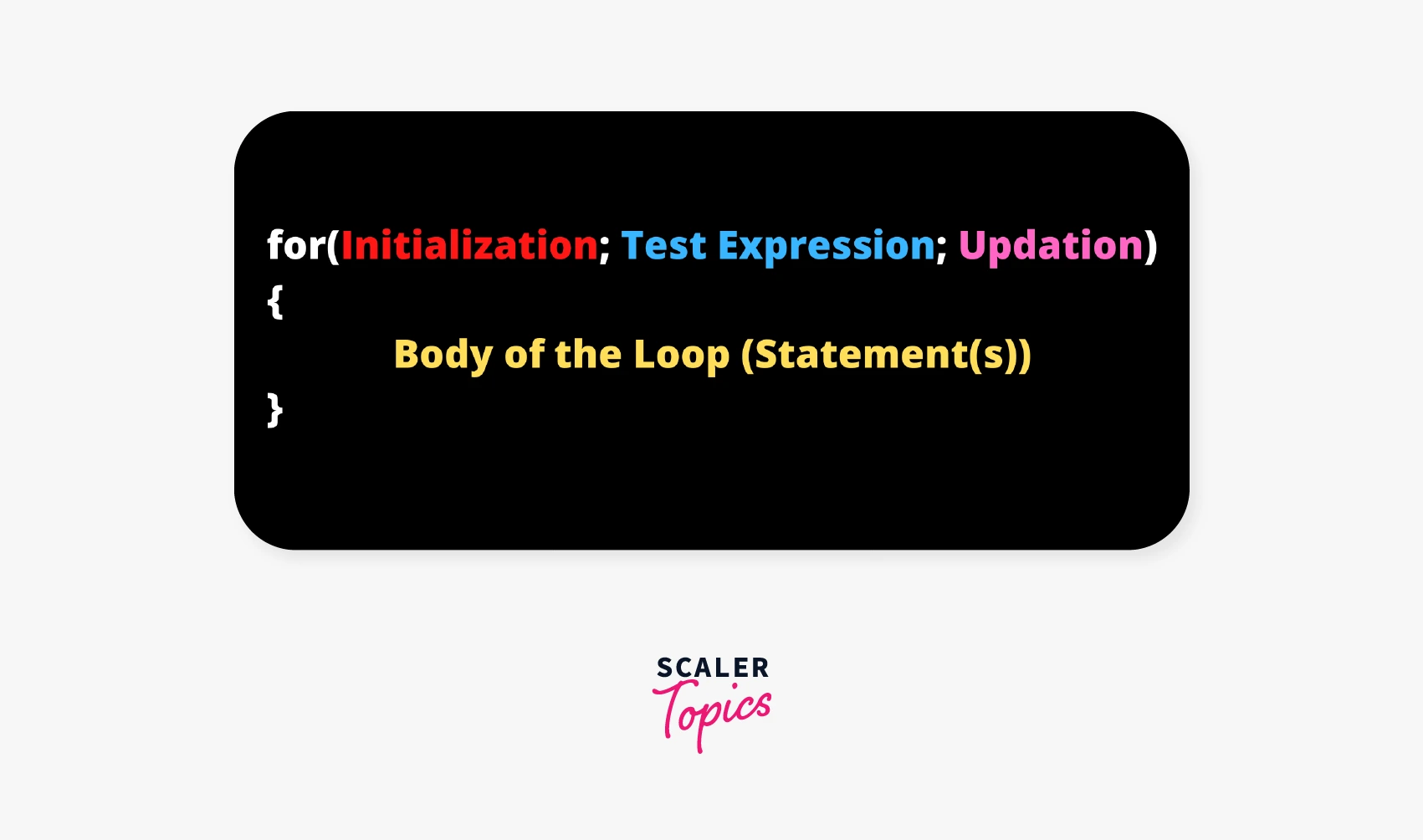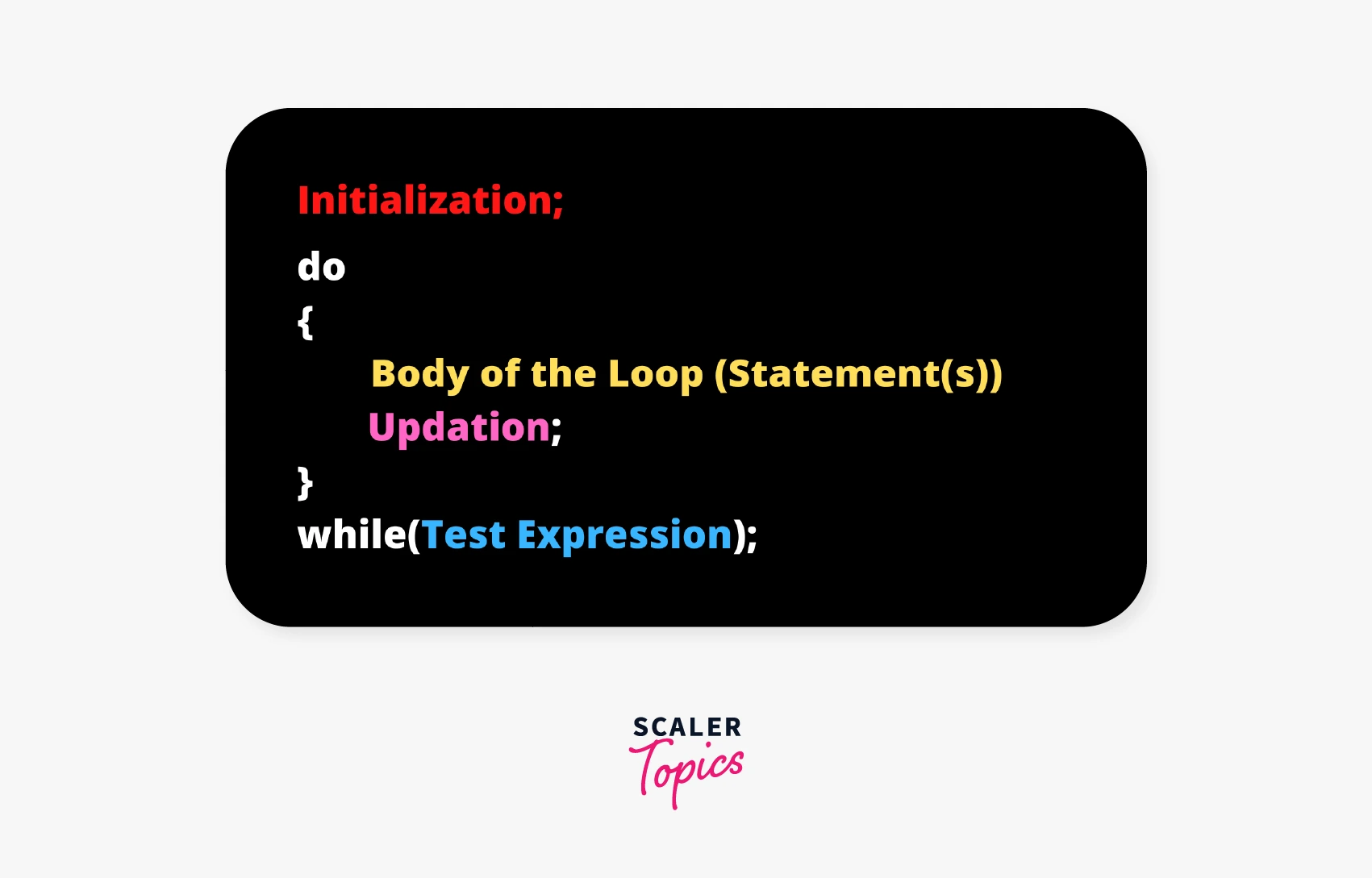## Conclusion

• Java provides three types of Loops: for, while, and do-while.
• Four Elements control a loop: initialization expression(s), test expression, loop-body, and update expression.
• While loop and for loop evaluates the test expression before allowing entry into the loop, hence are entry-controlled loops whereas, do-while is an exit-controlled loop as it evaluates the test expression after executing the body of the loop once.
• Loops can be made to run infinite times, they are called infinite loops. It is also possible to create an empty loop.
• We can create local variables inside the loop. Local variables are not visible outside the scope of its declaration.
• Loops also be initialized inside another loop. It is known as a nested loop.